# 14-1100/Homework Assignment 5

Jump to: navigation, search

This assignment is extended from class time on Wednesday, December 3, 2014 (a "virtual Monday" and the last day of the semester) to the end of Monday, December 8 in Dror's mailbox.

### Solve the following questions

Problem 1. Let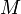$M$ be a module over a PID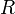$R$. Assume that$M$ is isomorphic to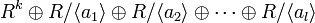$R^k\oplus R/\langle a_1\rangle\oplus R/\langle a_2\rangle\oplus\cdots\oplus R/\langle a_l\rangle$, with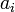$a_i$ non-zero non-units and with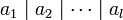$a_1\mid a_2\mid\cdots\mid a_l$. Assume also that$M$ is isomorphic to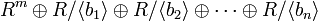$R^m\oplus R/\langle b_1\rangle\oplus R/\langle b_2\rangle\oplus\cdots\oplus R/\langle b_n\rangle$, with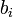$b_i$ non-zero non-units and with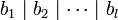$b_1\mid b_2\mid\cdots\mid b_l$. Prove that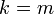$k=m$, that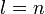$l=n$, and that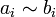$a_i\sim b_i$ for each$i$.

Problem 2. Let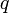$q$ and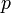$p$ be primes in a PID$R$ such that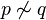$p\not\sim q$, let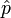$\hat{p}$ denote the operation of "multiplication by$p$", acting on any$R$-module$M$, and let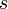$s$ and$t$ be positive integers.

1. For each of the$R$-modules$R$,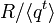$R/\langle q^t\rangle$, and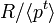$R/\langle p^t\rangle$, determine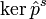$\ker\hat{p}^s$ and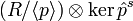$(R/\langle p\rangle)\otimes\ker\hat{p}^s$.
2. Explain why this approach for proving the uniqueness in the structure theorem for finitely generated modules fails.

Problem 3. (comprehensive exam, 2009) Find the tensor product of the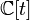${\mathbb C}[t]$ modules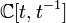${\mathbb C}[t,t^{-1}]$ ("Laurent polynomials in$t$") and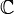${\mathbb C}$ (here$t$ acts on${\mathbb C}$ as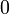$0$).

Problem 4. (from Selick) Show that if$R$ is a PID and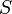$S$ is a multiplicative subset of$R$ then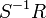$S^{-1}R$ is also a PID.

Definition. The "rank" of a module$M$ over a (commutative) domain$R$ is the maximal number of$R$-linearly-independent elements of$M$. (Linear dependence and independence is defined as in vector spaces).

Definition. An element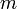$m$ of a module$M$ over a commutative domain$R$ is called a "torsion element" if there is a non-zero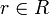$r\in R$ such that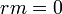$rm=0$. Let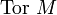$\mbox{Tor }M$ denote the set of all torsion elements of$M$. (Check that$\mbox{Tor }M$ is always a submodule of$M$, but don't bother writing this up). A module$M$ is called a "torsion module" if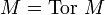$M=\mbox{Tor }M$.

Problem 5. (Dummit and Foote, page 468) Let$M$ be a module over a commutative domain$R$.

1. Suppose that$M$ has rank$n$ and that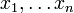$x_1,\ldots x_n$ is a maximal set of linearly independent elements of$M$. Show that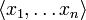$\langle x_1,\ldots x_n\rangle$ is isomorphic to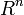$R^n$ and that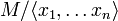$M/\langle x_1,\ldots x_n\rangle$ is a torsion module.
2. Conversely show that if$M$ contains a submodule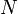$N$ which is isomorphic to$R^n$ for some$n$, and so that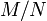$M/N$ is torsion, then the rank of$M$ is$n$.

Problem 6. (see also Dummit and Foote, page 469) Show that the ideal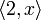$\langle 2,x\rangle$ in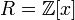$R={\mathbb Z}[x]$, regarded as a module over$R$, is finitely generated but cannot be written in the form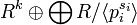$R^k\oplus\bigoplus R/\langle p_i^{s_i}\rangle$.